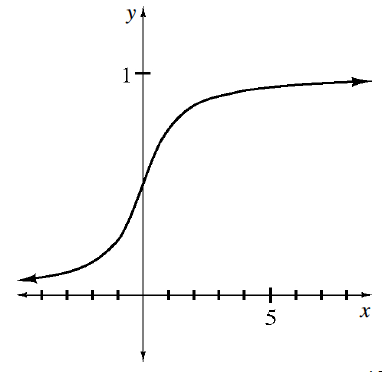### Home > CALC3RD > Chapter Ch9 > Lesson 9.4.3 > Problem9-129

9-129.

Given the graph of $y = f^\prime(x)$ below, draw a possible graph of $y = f(x)$. Why is there more than one possible solution? Homework Help ✎Your graph should have a slightly positive, but increasing, slope for $x < 0$.
It should have a slope of $≈ 1/2$ when $x = 0$.
The slope should increase for $x > 0$, but not exceed $1$ .